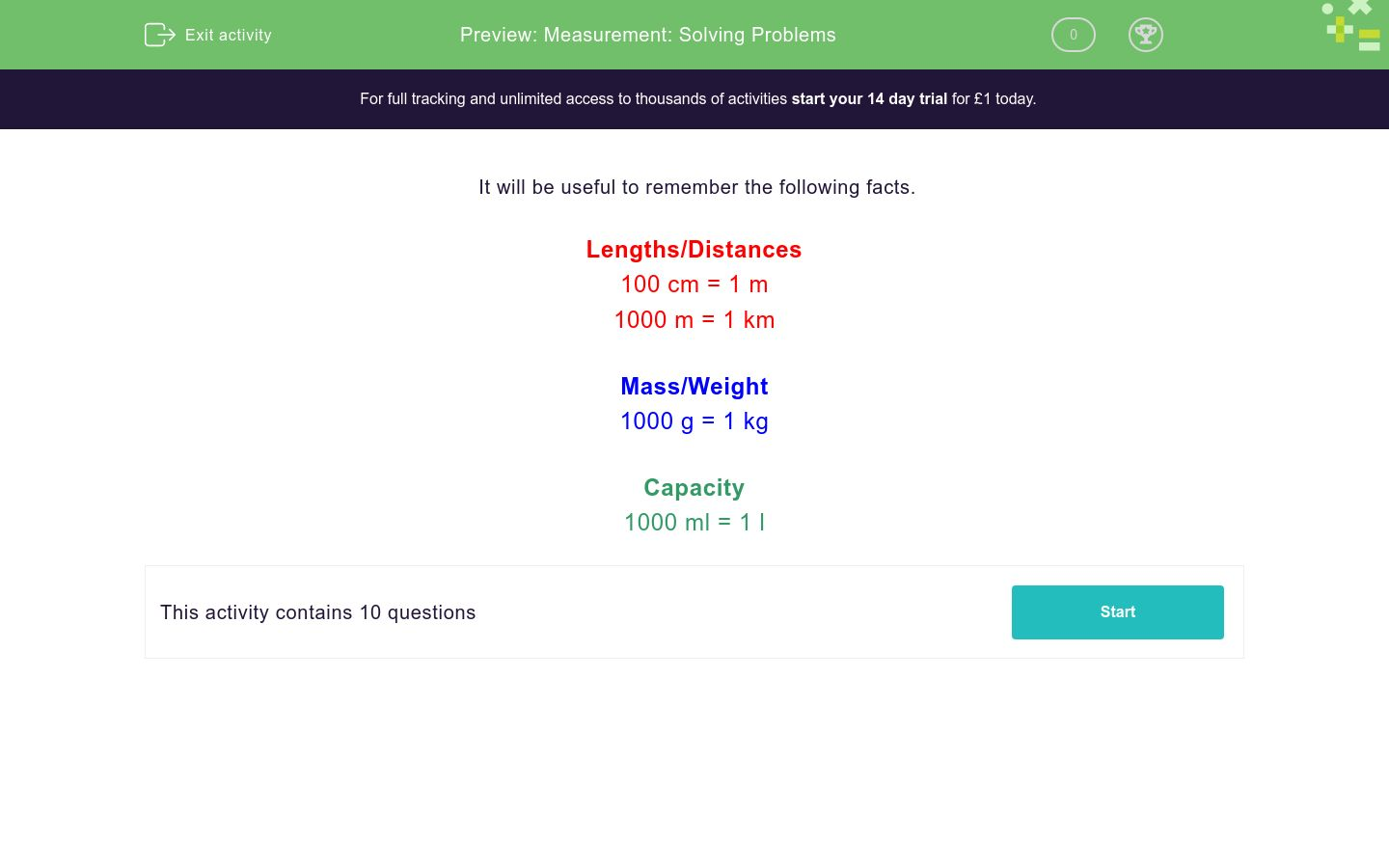# Measurement: Solving Problems

In this worksheet, students answer a selection of word problems relating to measurements.Key stage:  KS 2

Curriculum topic:   Measurement

Curriculum subtopic:   Convert Units of Measure

Difficulty level:### QUESTION 1 of 10

It will be useful to remember the following facts.

Lengths/Distances

100 cm = 1 m

1000 m = 1 km

Mass/Weight

1000 g = 1 kg

Capacity

1000 ml = 1 l

It is 2 km from my house to Uncle Jack's house.

How many metres is this?

Just write the number.

Last Sunday, I went for a 6½ km walk.

How many metres is this?

Just write the number.

One side of a square is 20 cm long

How many centimetres is it all the way round the square?

Just write the number.

Jack is 140 cm tall. Jill is 1½ m tall.

Who is taller?

How many grams are there in a 1 kilogram bag of flour?

Just write the number.

How many grams are there in a ¼ kilogram packet of butter?

Just write the number.

Amy buys ½ kg of apples and 1000 g of cherries.

How much does this fruit weigh altogether?

1000½ g

1500 g

1.5 kg

1½ kg

Amy weighs 35 kg of apples and her hamster weighs 1000 g.

How much do they both weigh altogether?

1035 g

35000 g

36 kg

36000 g

A can of coke holds 330 ml.

Roughly how many cans make 1 litre?

Just write the number.

When half full, a bucket holds 4500 ml.

How many litres does it hold when it is completely full?

Just write the number.

• Question 1

It is 2 km from my house to Uncle Jack's house.

How many metres is this?

Just write the number.

2000
EDDIE SAYS
1000 m = 1 km 2 x 1000 = 2000
• Question 2

Last Sunday, I went for a 6½ km walk.

How many metres is this?

Just write the number.

6500
EDDIE SAYS
1000 m = 1 km 6.5 x 1000 = 6500
• Question 3

One side of a square is 20 cm long

How many centimetres is it all the way round the square?

Just write the number.

80
EDDIE SAYS
20 x 4 = 80
• Question 4

Jack is 140 cm tall. Jill is 1½ m tall.

Who is taller?

Jill
EDDIE SAYS
100 cm = 1 m 100 + 50 = 150 Jill is taller.
• Question 5

How many grams are there in a 1 kilogram bag of flour?

Just write the number.

1000
EDDIE SAYS
1000 g = 1 kg
• Question 6

How many grams are there in a ¼ kilogram packet of butter?

Just write the number.

250
EDDIE SAYS
1000 g = 1 kg 1000/4 = 250
• Question 7

Amy buys ½ kg of apples and 1000 g of cherries.

How much does this fruit weigh altogether?

1500 g
1.5 kg
1½ kg
EDDIE SAYS
1000 g = 1 kg 1000 + 500 = 1500 g, 1.5 kg, 1 1/2 kg
• Question 8

Amy weighs 35 kg of apples and her hamster weighs 1000 g.

How much do they both weigh altogether?

36 kg
36000 g
EDDIE SAYS
1000 g = 1 kg 35 x 1000 = 35000 35000 + 1000 = 36000 g, 36 kg
• Question 9

A can of coke holds 330 ml.

Roughly how many cans make 1 litre?

Just write the number.

3
EDDIE SAYS
1000 ml = 1 l 1000/330 = 3.03, so about 3 cans make 1 l
• Question 10

When half full, a bucket holds 4500 ml.

How many litres does it hold when it is completely full?

Just write the number.

9
EDDIE SAYS
1000 ml = 1 l 4500 x 2 = 9000 9000/1000 = 9 l
---- OR ----

Sign up for a £1 trial so you can track and measure your child's progress on this activity.

### What is EdPlace?

We're your National Curriculum aligned online education content provider helping each child succeed in English, maths and science from year 1 to GCSE. With an EdPlace account you’ll be able to track and measure progress, helping each child achieve their best. We build confidence and attainment by personalising each child’s learning at a level that suits them.

Get started